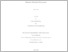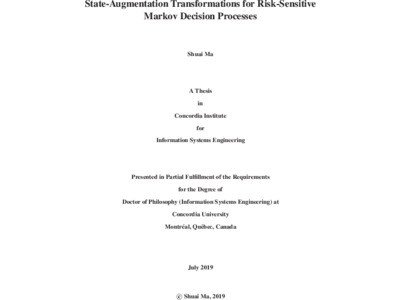Title:

# State-Augmentation Transformations for Risk-Sensitive Markov Decision Processes

Ma, Shuai (2019) State-Augmentation Transformations for Risk-Sensitive Markov Decision Processes. PhD thesis, Concordia University.Preview
Text (application/pdf)
Ma_PhD_S2020.pdf - Accepted Version
1MB

## Abstract

Markov decision processes (MDPs) provide a mathematical framework for modeling sequential decision making (SDM) where system evolution and reward are partly under the control of a decision maker and partly random. MDPs have been widely adopted in numerous fields, such as finance, robotics, manufacturing, and control systems. For stochastic control problems, MDPs serve as the underlying models in dynamic programming and reinforcement learning (RL) algorithms.

In this thesis, we study risk estimation in MDPs, where the variability of random rewards is taken into account. First, we categorize the reward into four classes: deterministic/stochastic and state-/transition-based. Though numerous of theoretical methods are designed for MDPs or Markov processes with a deterministic (and state-based) reward, many practical problems are naturally modeled by processes with stochastic (and transition-based) reward. When the optimality criterion refers to the risk-neutral expectation of a (discount) total reward, we can use a model (reward) simplification to bridge the gap. However, when the criterion is risk-sensitive, a model simplification will change the risk value. For preserving the risks, we address that most, if not all, the inherent risk measures depend on the reward sequence (Rt). In order to bridge the gap between theoretical methods and practical problems with respect to risk-sensitive criteria, we propose a state-augmentation transformation (SAT). Four cases are thoroughly studied in which different forms of SAT should be implemented for risk preservation. In numerical experiments, we compare the results from the model simplifications and the SAT, and illustrate that, i). the model simplifications change (Rt) as well as return (or total reward) distributions; and ii). the proposed SAT transforms processes with complicated rewards, such as stochastic and transition-based rewards, into ones with deterministic state-based rewards, with intact (Rt).

Second, we consider constrained risk-sensitive SDM problems in dynamic environments. Unlike other studies, we simultaneously consider the three factors—constraint, risk, and dynamic environment. We propose a scheme to generate a synthetic dataset for training an approximator. The reasons for not using historical data are two-fold. The first reason refers to information incompleteness. Historical data usually contains no information on criterion parameters (which risk objective and constraint(s) are concerned) and (or) the optimal policy (usually just an action for each item of data), and in many cases, even the information on environmental parameters (such as all involved costs) is incomplete. The second reason is about optimality. The decision makers might prefer an easy-to-use policy than an optimal one, which is hard to determine whether the preferred policy is optimal (such as an EOQ policy), since the practical problems could be different from the theoretical model diversely and subtly. Therefore, we propose to evaluate or estimate risk measures with RL methods and train an approximator, such as neural network, with a synthetic dataset. A numerical experiment validates the proposed scheme.

The contributions of this study are three-fold. First, for risk evaluation in different cases, we propose the SAT theorem and corollaries to enable theoretical methods to solve practical problems with a preserved (Rt). Second, we estimate three risk measures with return variance as examples to illustrate the difference between the results from the SAT and the model simplification. Third, we present a scheme for constrained, risk-sensitive SDM problems in a dynamic environment with an inventory control example.

Divisions: Concordia University > Gina Cody School of Engineering and Computer Science > Concordia Institute for Information Systems Engineering Thesis (PhD) Ma, Shuai Concordia University Ph. D. Information and Systems Engineering July 2019 Yu, Jia Yuan and Satir, Ahmet 986039 SHUAI MA 25 Jun 2020 18:24 25 Jun 2020 18:24
All items in Spectrum are protected by copyright, with all rights reserved. The use of items is governed by Spectrum's terms of access.

Repository Staff Only: item control pageResearch related to the current document (at the CORE website)
Back to top